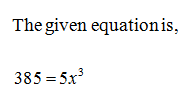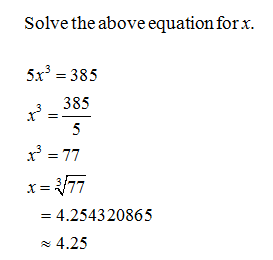# Solve for x.385=5x3Express the answer to the hundredths place (i.e., two digits after the decimal point).x=

Question
1 views
Solve for x.

385=5x3

Express the answer to the hundredths place (i.e., two digits after the decimal point).

x=
check_circle

Step 1Step 2...

### Want to see the full answer?

See Solution

#### Want to see this answer and more?

Solutions are written by subject experts who are available 24/7. Questions are typically answered within 1 hour.*

See Solution
*Response times may vary by subject and question.
Tagged in